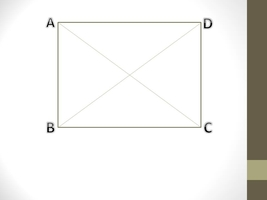# The length of a rectangle is 5 yd more than twice its width and area of the rectangle is 42 yd...

## Question:

The length of a rectangle is {eq}5 \;yd {/eq} more than twice its width and area of the rectangle is {eq}42 \;yd ^2 {/eq}, find the dimensions.

## Rectangle Area and Properties

A quadrilateral with opposite and parallel sides of equal length is called a rectangle.

In a rectangle, all interior angles are right angles means all angles are of {eq}90^{\circ}. {/eq}A rectangle has two diagonals of equal length.

#### Area of a Rectangle

{eq}\displaystyle A = L \times W {/eq}

Here {eq}A = {/eq} area

{eq}L = {/eq} length

{eq}W = {/eq} width of the rectangle

given-

The length of the rectangle is 5 yds more than twice its width.

{eq}\displaystyle L = 5 + 2W -----------(1) {/eq}

Also given that area of the rectangle is {eq}42 yds ^{2} {/eq}

{eq}\displaystyle A = 42 yd^{2} {/eq}

{eq}\displaystyle L \times W = 42 {/eq}

from eqution(1)-

{eq}\displaystyle (5+2W)W = 42 {/eq}

{eq}\displaystyle 2W^{2} +5W -42 = 0 {/eq}

{eq}\displaystyle W = \frac{-5 \pm \sqrt{5^{2}-4 \times 2 \times (-42)}}{2 \times 2} {/eq}

{eq}\displaystyle W = \frac{-5 \pm \sqrt{25+336}}{4} {/eq}

{eq}\displaystyle W = \frac{-5 \pm \sqrt{361}}{4} {/eq}

{eq}\displaystyle W = \frac{-5 \pm 19 }{4} {/eq}

so {eq}\displaystyle W = \frac{-5 + 19 }{4} = \frac{7}{2} = 3.5 {/eq}

and {eq}\displaystyle W = \frac{-5 - 19 }{4} = -6 {/eq}

Because the width of a rectangle can not be negative so {eq}\displaystyle W = -6 {/eq} is unacceptable.

So

{eq}\displaystyle W = \frac{7}{2} {/eq}

from equation(1)-

{eq}\displaystyle L = 5 + 2 \times \frac{7}{2} {/eq}

{eq}\displaystyle L = 5+7 = 12 {/eq}

So the length and width of the rectangle are 12 yds and 3.5 yds respectively.